# What I Learned while working on an Elixir AppAtul Bhosale

Recently, I was working on writing a background worker using Elixir for one of our clients. This post is about what I learned while writing the worker.

I tried addition for Decimal type, the same way as we do Integers like: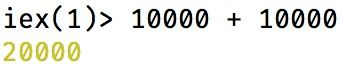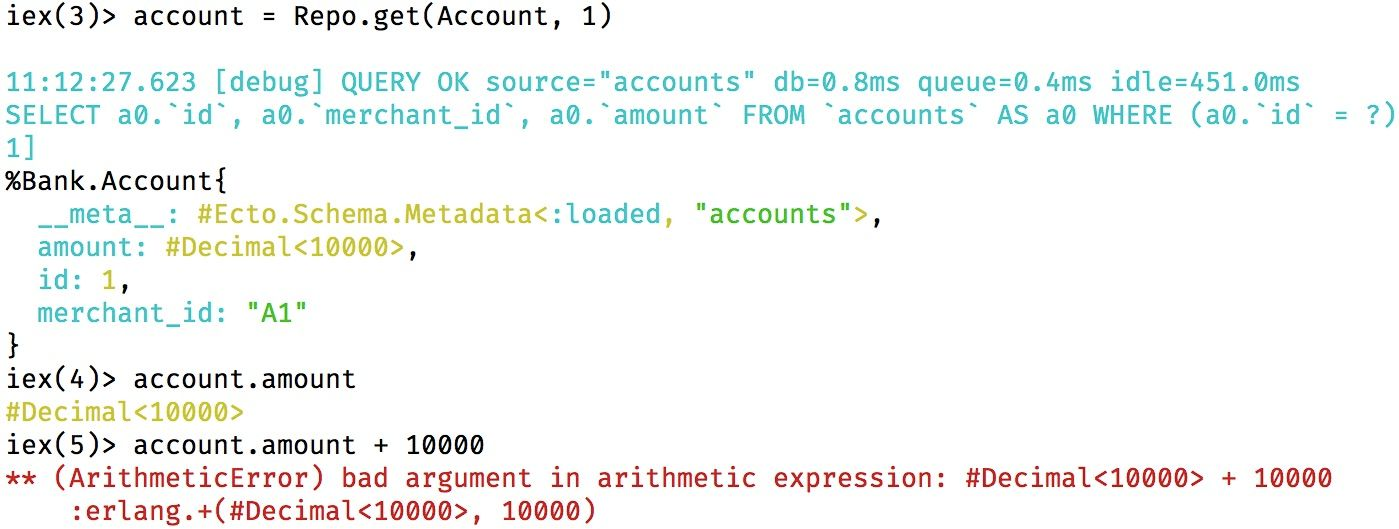It raises an Arithmetic error since Decimal has an underlying struct:

``````[%Decimal{coef: coefficient(), exp: exponent(), sign: sign()}][decimal-struct]
``````

I checked the decimal library & I found that it has an add function to add Decimal or integer values. Hence the addition can be done as follows: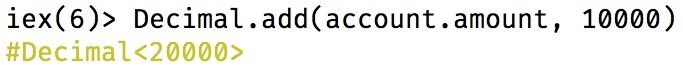# Comparing decimal values

Integer comparison can be done with `==` operator.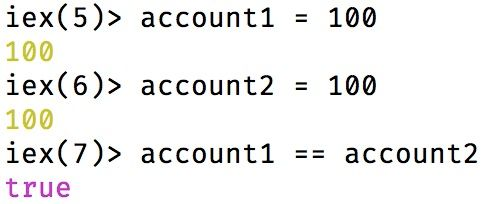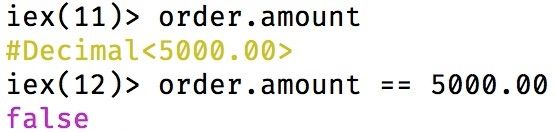It returns false since the value on RHS(Right-hand-side) of `==` operator is not a decimal.

I tried using Decimal.new as follows: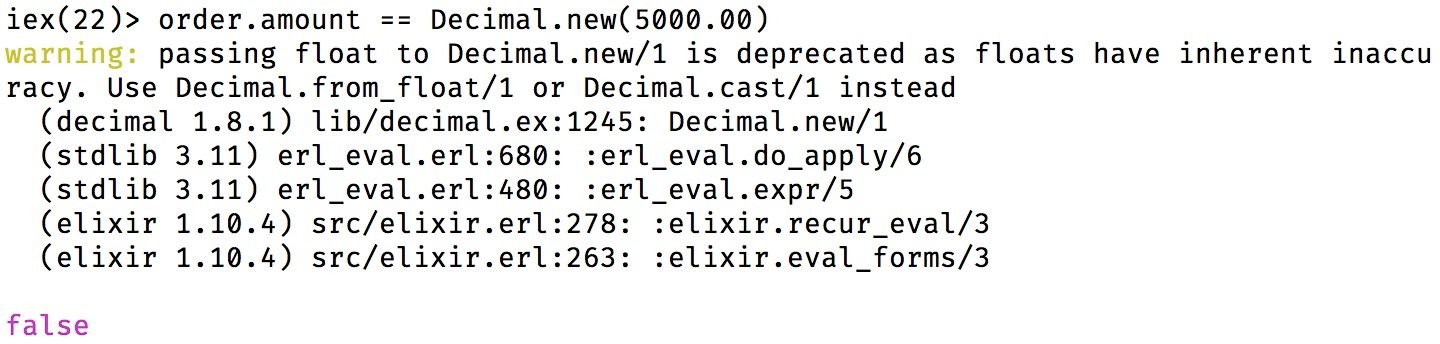Based on the warning message I tried using Decimal.from_float & Decimal.cast as follows: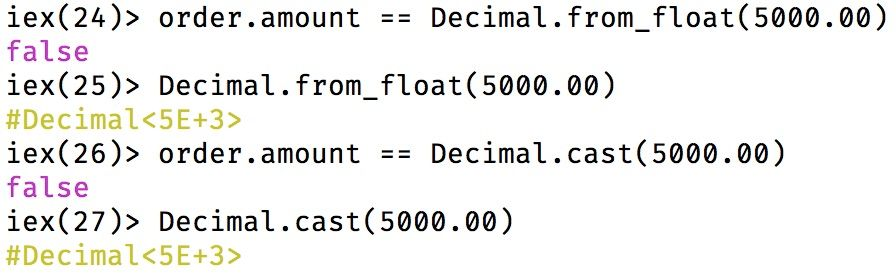but that too returns false.

I then tried to pass a string to Decimal.new as follows: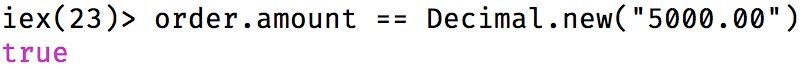and it worked.

# Approach using find_in_batches

While working on a business use case to fetch the pending orders & to process them. The code would be as follows:

``````  defp orders_query() do
from(order in Order,
where: order.status == ^@pending
)
end

def perform do
orders_query()
|> Repo.all()
|> Enum.each(fn order ->
update_order(order)
end)
end
``````

The above code will load all the records at once in the memory. I decided to search for something which will be similar to find_in_batches from Rails in Elixir and I found this discussion.

The updated code will be as follows:

``````  def perform do
Repo.transaction(fn ->
orders_query()
|> Repo.stream()
|> Enum.each(fn order ->
update_order(order)
end)
end)
end
``````

I have used Repo.stream which by default fetches the records in batches of 500 & it needs to be wrapped in a transaction.

# Recursion

In the above approach, I faced an issue. When the time taken to update the records exceeds the timeout, Ecto would raise a timeout error which I have described here.

I solved it by using recursion as follows:

``````  @batch_size 500

def perform do
remaining_records_count()
|> iterate_multiple_times()
end

defp remaining_records_count do
orders_query()
|> Repo.aggregate(:count)
end

defp iterate_multiple_times(count) when count <= @batch_size,
do: make_account_balance_available()

defp iterate_multiple_times(_count) do
make_account_balance_available()

remaining_records_count()
|> iterate_multiple_times()
end
``````

In the above code, the `iterate_multiple_times/1` is a recursive function which calls itself until there aren't any remaining records.

I hope you will find these learnings helpful while building any app/library using Elixir.